# Statistics - math word problems

#### Number of problems found: 278

• Three groupsIn the company, employees are divided into three groups. In the first group, which includes 12% of the company's total number of employees, the average salary is CZK 40,000, in the second group CZK 35,000, in the third group CZK 25,000. The average salary
• Trapezoid 25Trapezoid PART with AR||PT has (angle P=x) and (angle A=2x) . In addition, PA = AR = RT = s. Find the length of the median of Trapezoid PART in terms of s.
• Find the meanFind the number between 13 and 29.
• Tennis aces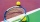The number of aces served by Novak Djokovic in the last 20 tournaments that he has participated in, is shown below. 12 17 13 7 8 14 11 14 10 12 15 9 11 13 6 15 18 5 19 24 1.1 using the raw, determine the range. 1.2 Group the data into a frequency distribu
• Convalescent homes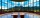In 270 convalescent homes, 94,270 vacationers spent part of the holiday a year. On average, how many vacationers per convalescent home?
• Playing cards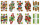From 32 playing cards containing 8 red cards, we choose 4 cards. What is the probability that just 2 will be red?
• Coordinates of the intersection of the diagonals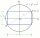In the rectangular coordinate system, a rectangle ABCD is drawn. The vertices of the rectangle are determined by these coordinates A = (2.2) B = (8.2) C = (8.6) D = (2.6) Find the coordinates of the intersection of the diagonals of the ABCD rectangle
• Beer tapping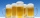When checking compliance with the beer tapping, it was found that 60% of the offered beers were underfilled. The others were fine. Thus, instead of 0.5 l, the volume was 4.4 dcl on average. What was the volume of one average underfilled beer?
• Six questions testThere are six questions in the test. There are 3 answers to each - only one is correct. In order for a student to take the exam, at least four questions must be answered correctly. Alan didn't learn at all, so he circled the answers only by guessing. What
• Water mixingWe have 520 ml of hot water and 640 ml of water at 48°C. What is the temperature of approximately hot water when the resulting mixture has a temperature of 65°C?
• Average priceSixth grade went on a trip to Moravia. Each of the 26 pupils paid CZK 320 and the school paid a total of CZK 3,510. What was the average price of a trip per student?
• Probability of failures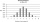In certain productions, the probability of failures is 0.01. Calculate the probability that there will be more than 1 failure among the 100 selected products if we return the selected products to the file after the check.
• All use computerIt is reported that 72% of working women use computers at work. Choose 3 women at random, find the probability that all 3 women use a computer in their jobs.
• Average heightThere are twice as many girls in the class as there are boys. The average height of girls is 177 cm, boys 186 cm. What is the average height of students in this class?
• My fatherMy father cut 78 slats on the fence. The shortest of them was 97 cm long, the longer one was 102 cm long. What was the total length of the slats in cm?
• Coordinates hexagon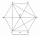The regular hexagon ABCDEF is given. Point A has coordinates [1; 3] and point D has coordinates [4; 7]. Calculate the sum of the coordinates of the center of its described circle.
• Large familyThe average age of all family members (children, mother, father, grandmother, grandfather) is 29 years. The average age of parents is 40 years, grandparents 66 years and all children are 5 years. How many children are there in this family?
• The testThe test contains four questions, and there are five different answers to each of them, of which only one is correct, the others are incorrect. What is the probability that a student who does not know the answer to any question will guess the right answer
• Medians in RT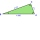The rectangular triangle ABC has a length of 10 cm and 24 cm. Points P, Q, R are the centers of the sides of this triangle. The perimeter of the PQR triangle is:
• TroopsThere are two leaders in the tourist group, with an average age of 30 years and several children with an average age of 10 years. The total age of the group is 12 years. How many children are in the group?

Do you have an interesting mathematical word problem that you can't solve it? Submit a math problem, and we can try to solve it.

We will send a solution to your e-mail address. Solved examples are also published here. Please enter the e-mail correctly and check whether you don't have a full mailbox.

Please do not submit problems from current active competitions such as Mathematical Olympiad, correspondence seminars etc...